#### Vector fields

A vector field looks like a needle diagram'' over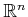, as depicted in Figure 8.5. The idea is to specify a direction at each point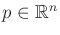. When used to represent a feedback plan, it indicates the direction that the robot needs to move if it finds itself at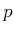.

For every, associate an-dimensional vector space called the tangent space at, which is denoted as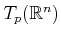. Why not just call it a vector space at? The use of the word tangent'' here might seem odd; it is motivated by the generalization to manifolds, for which the tangent spaces will be tangent'' to points on the manifold.

A vector field8.4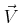onis a function that assigns a vector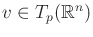to every. What is the range of this function? The vector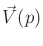at eachactually belongs to a different tangent space. The range of the function is therefore the union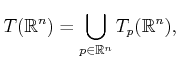(8.9)

which is called the tangent bundle on. Even though the way we describe vectors frommay appear the same for any, each tangent space is assumed to produce distinct vectors. To maintain distinctness, a point in the tangent bundle can be expressed with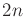coordinates, by specifyingand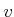together. This will become important for defining phase space concepts in Part IV. In the present setting, it is sufficient to think of the range ofasbecause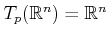for every.

A vector field can therefore be expressed usingreal-valued functions on. Let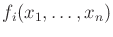forfromtodenote such functions. Using these, a vector field is specified as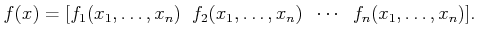(8.10)

In this case, it appears that a vector field is a function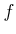frominto. Therefore, standard function notation will be used from this point onward to denote a vector field.

Now consider some examples of vector fields over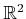. Let a point inbe represented as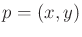. In standard vector calculus, a vector field is often specified as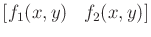, in which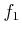and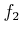are functions on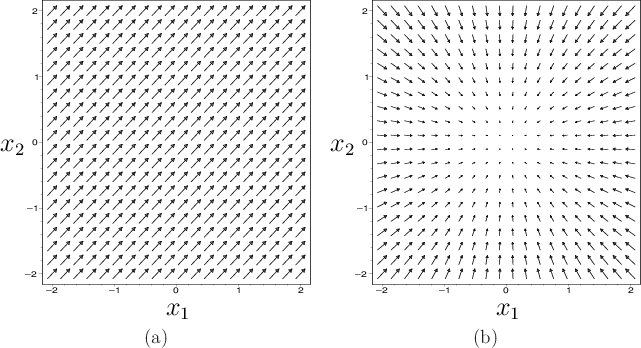Example 8..6 (Constant Vector Field)   Figure 8.5a shows a constant vector field, which assigns the vector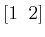to every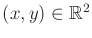.Example 8..7 (Inward Flow)   Figure 8.5b depicts a vector field that assignsto every. This causes all vectors to point to the origin.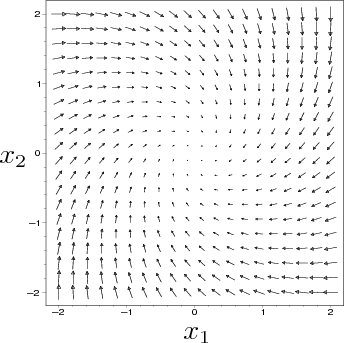Example 8..8 (Swirl)   The vector field in Figure 8.6 assigns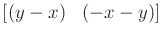to every.Due to obstacles that arise in planning problems, it will be convenient to sometimes restrict the domain of a vector field to an open subset of. Thus, for any open subset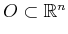, a vector field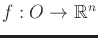can be defined.

Steven M LaValle 2020-08-14